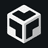# Understanding type compatibility

In this section, we'll learn how TypeScript decides whether an item can be assigned to another. i.e., how TypeScript decides whether types are compatible.

## Basic type compatibility

Let's explore type compatibility in an exercise.Open exercise in CodeSandbox

The code sets a string variable to the value of a number variable. Hopefully, it is no surprise that TypeScript isn't happy with the assignment on the last line because the types aren't compatible.

• Now enter the following code into the editor:
`.css-wr5eo0{position:absolute;top:0;right:0;z-index:1;border-radius:0 5px 0 5px;padding:0.25rem 0.6rem;border:none;cursor:pointer;background:#44475a;color:rgb(248,248,242);-webkit-transition:all 200ms ease;transition:all 200ms ease;font-size:12px;}.css-wr5eo0:disabled{cursor:not-allowed;}.css-wr5eo0:not(:disabled):hover,.css-wr5eo0:not(:disabled):focus{background:#3b3b5d;}`let jones: "Tom" | "Bob" = "Tom";let jane: string = "Jane";jane = jones;``
🤔

Is TypeScript happy with the assignment on line 3?

• What about if we switch the assignment around:
``jones = jane;``
🤔

Is TypeScript happy with the assignment now?

If type, `A`, is a subset of another type, `B`, a value in type `A` can be assigned to a variable of type `B`. However, a value in type `B` can't be assigned to a variable of type `A`.

## Object type compatibility

TypeScript is a structurally-typed language, which means types are based only on their members. Let's explore this.

• Copy and paste the following code into the editor:
``type Person = {  name: string;};interface IPerson {  name: string;}let bob: Person = {  name: "Bob",};let fred: IPerson = {  name: "Fred",};bob = fred;``

The types `Person` and `IPerson` below are equivalent because the type members are the same:

The type names aren't important in TypeScript type compatibility - it is the structure that is important.

• Let's add an `age` property to `IPerson`
``type Person = {  name: string;};interface IPerson {  name: string;  age: number;}let bob: Person = {  name: "Bob",};let fred: IPerson = {  name: "Fred",  age: 30,};bob = fred;``
🤔

Is the assignment on line 17 okay now?

• Move the `age` property to `Person`:
``type Person = {  name: string;  age: number;};interface IPerson {  name: string;}let bob: Person = {  name: "Bob",  age: 30,};let fred: IPerson = {  name: "Fred",};bob = fred;``
🤔

Is the assignment okay now?

• Let's try a different example:
``type Dog = {  name: string;};type Shape = {  name: "Circle" | "Square";};let ben: Dog = {  name: "Ben",};let circle: Shape = {  name: "Circle",};circle = ben;``
🤔

Will the assignment raise a type error on line 13?

The types of the members of the objects are essential. Each member type has to be compatible for the object to be compatible.

• Let's switch the assignment around:
``type Dog = {  name: string;};type Shape = {  name: "Circle" | "Square";};let ben: Dog = {  name: "Ben",};let circle: Shape = {  name: "Circle",};ben = circle;``

{" "}

🤔

Is the assignment going to be okay now?

## Function type compatibility

Type compatibility for functions is based on structure as well. TypeScript checks that the function parameter types and the return type are compatible. Let's explore this:

• Copy and paste the code below into the code editor:
``let add = (a: number, b: number): number => a + b;let sum = (x: number, y: number): number => x + y;sum = add;``
🤔

Is the assignment on line 3 okay?

The parameter names aren't important - it is only the types of parameters that are checked for type compatibility.

• Let's introduce an additional parameter into the `add` function:
``let add = (a: number, b: number, c: number): number => a + b + c;let sum = (x: number, y: number): number => x + y;sum = add;``
🤔

Is the assignment okay now?

So, the number of parameters is important.

• Let's make the `c` parameter optional:
``let add = (a: number, b: number, c?: number): number => a + b + (c || 0);let sum = (x: number, y: number): number => x + y;sum = add;``
🤔

Is the assignment ok now?

• Let's make `c` required again and switch the assignment around:
``let add = (a: number, b: number, c: number): number => a + b + c;let sum = (x: number, y: number): number => x + y;add = sum;``
🤔

Is the assignment ok now?

If function parameters are a subset of the parameters of another function, it can be assigned to it.

## Summary

TypeScript uses structural typing, which means that variables with different types can be assigned to one another if the types are compatible. Here are some rules we can use to determine whether types are compatible:

• A variable, `a`, can be assigned to another variable, `b`, if the type of `b` is wider than the type of `a`.
• An object, `a`, can be assigned to another object, `b`, if `a` has at least the same members as `b`.
• A function, `a`, can be assigned to another function, `b`, if each parameter in `a` has a corresponding parameter in `b` with a compatible type.

Next, let’s check what we have learned with a quiz.